• 一个简单的例子从最简单的情况出发,连续可微的封闭曲线为边界的,最简单例子是”圆”. ——“什么？你忽悠我？！” 为了不至于激起不满，我临时决定把这个简单的例子更改为椭圆。当然，如果椭圆的长短轴跟直角坐标...
先把标题放在这里,内容我慢慢准备,一点点添加1.

一个简单的例子

从最简单的情况出发,连续可微的封闭曲线为边界的,最简单例子是”圆”.
——“什么？你忽悠我？！”
为了不至于激起不满，我临时决定把这个简单的例子更改为椭圆。当然，如果椭圆的长短轴跟直角坐标系的坐标轴平行，其实也还简单，所以让它再旋转一个角度θ<!--//--><![CDATA[//><!--
\theta
//--><!]]>。所以我选择了如下参数方程的椭圆： {x=y=5costcosθ−3sintsinθ3cosθsint+5costsinθ<!--//--><![CDATA[//><!--
\left\{\;
\begin{array}{rl}
x=&5 \cos t \cos \theta -3 \sin t \sin \theta  \\
y=&3 \cos \theta  \sin t+5 \cos t \sin \theta \\
\end{array}
\right.
//--><!]]>为了把曲线画出来, 让θ=30o<!--//--><![CDATA[//><!--
\theta=30^o
//--><!]]>, 从而其参数方程变为: ⎧⎩⎨⎪⎪⎪⎪x=y=12(53√cost−3sint)12(5cost+33√sint)<!--//--><![CDATA[//><!--
\left\{\;
\begin{array}{rl}
x=&\frac{1}{2} \left(5 \sqrt{3} \cos t-3 \sin t\right) \\
y=&\frac{1}{2} \left(5 \cos t+3 \sqrt{3} \sin t\right) \\
\end{array}
\right.
//--><!]]>ParametricPlot[RotationTransform[Pi/6][curve], {t, 0, 2 Pi}] /.  Line[x_] :> {Red, Line[x]}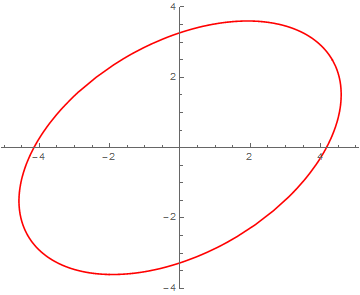要判断任给点P=(x0,y0)<!--//--><![CDATA[//><!--
P=(x_0,y_0)
//--><!]]>是不是在图中的浅蓝色区域: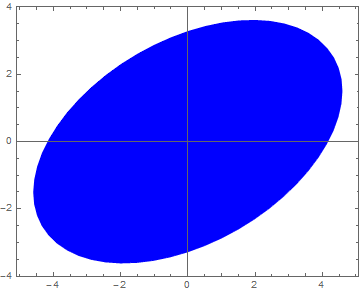不知道常用的都是什么办法? 2.
.
至少对圆的情形,判断的方法是到圆心的距离跟圆的半径作比较. 但是椭圆不同了,而且旋转了θ<!--//--><![CDATA[//><!--
\theta
//--><!]]>角,让自然参数t<!--//--><![CDATA[//><!--
t
//--><!]]>也不直接对应于P<!--//--><![CDATA[//><!--
P
//--><!]]>点的方位了,只是稍微增加了一点点麻烦而已,仍然可以找出椭圆上的边界点,然后对比到椭圆圆心的距离. 这个方法太原始了,而且不易推广,显然不是我想说的.

看看math.stackexchange.com上人家怎么说吧.如何确定任一点是否在二次曲线内,我当然不是为了转一个链接而卖关子. 链接里的方法主要还是对方程为隐函数g(x,y)=Ax2+Bxy+Cy2+Dx+Ey+F=0<!--//--><![CDATA[//><!--
g(x,y) = Ax^2+Bxy+Cy^2+Dx+Ey+F=0
//--><!]]>形式的二次曲线,利用其二次型.但仅适用于二次曲线.

大部分情况下,参数方程更方便,而且两种形式之间互换本来也挺麻烦. 这里介绍基于椭圆的参数方程时,如何判断; 同时,把这个例子里的概念推广到更一般的高于二次的C∞<!--//--><![CDATA[//><!--
C^\infty
//--><!]]>曲线，以及凸的和凹的多边形.

这个概念就是拓扑学中的winding number 或 卷绕数3.

很容易把任意曲线的参数方程: {x=y=x(t)y(t)<!--//--><![CDATA[//><!--
\left\{\;
\begin{array}{rl}
x=& x(t) \\
y=& y(t) \\
\end{array}
\right.
//--><!]]>
改写成极坐标形式: ⎧⎩⎨⎪⎪⎪⎪⎪⎪ρ=θ=x(t)2+y(t)2−−−−−−−−−−√arctan(y(t)x(t)) 特殊的反正切,应该带卦限信息,并兼容x(t)=0<!--//--><![CDATA[//><!--
\left\{\;
\begin{array}{rl}
\rho =& \sqrt{x(t)^2+y(t)^2} \\
\theta=& \arctan\left(\dfrac{y(t)}{x(t)}\right) \text{ 特殊的反正切,应该带卦限信息,并兼容}x(t)=0\\
\end{array}
\right.
//--><!]]>求导数之后(参考维基上的内容winding number 或 卷绕数):

dθ=x(t)y′(t)−y(t)x′(t)x(t)2+y(t)2dt<!--//--><![CDATA[//><!--
d\theta=\dfrac{x(t) y'(t)-y(t) x'(t)}{x(t)^2+y(t)^2}dt
//--><!]]>

曲线x(t)−y(t)<!--//--><![CDATA[//><!--
x(t)-y(t)
//--><!]]>在某点(x0,y0)<!--//--><![CDATA[//><!--
(x_0,y_0)
//--><!]]>的卷绕数定义为: w(x0,y0)=12π∫t1t0(x(t)−x0)y′(t)−(y(t)−y0)x′(t)(x(t)−x0)2+(y(t)−y0)2dt<!--//--><![CDATA[//><!--
\text{w}_{(x_0,y_0)}=\dfrac{1}{2\pi}\int_{t_0}^{t_1}\frac{(x(t)-{x_0}) y'(t)-(y(t)-{y_0})x'(t)}{\left(x(t)-{x_0}\right)^2+\left(y(t)-{y_0}\right)^2}dt
//--><!]]>
对于前面提到的椭圆:t0=0,t1=2π<!--//--><![CDATA[//><!--
t_0=0, t_1=2\pi
//--><!]]>,**当w(x0,y0)<!--//--><![CDATA[//><!--
\rm{w}(x_0,y_0)
//--><!]]>为0时,点在椭圆外,为非0整数时在其内。

这个结论使得用数值积分计算结果作为判据非常合适,因为它对积分的数值计算有很大的允许误差; 从维基还是某本书上提到,winding number在量子力学里面又是一种”量子数”(稍后核实出处)。

用Mathematica代码做个试验:

curve={5Cos[t],3Sin[t]};  (*标准椭圆定义*)
{x[t],y[t]}=RotationTransform[Pi/6]@curve; (*旋转30°的参数形式*)
innerQ[{x0_,y0_}]:=Round[NIntegrate[((x[t]-x0)D[y[t],t]-(y[t]-y0)D[x[t],t])/((x[t]-x0)^2+(y[t]-y0)^2),{t,0,2Pi}]/2/Pi]!=0 (*以卷绕数为判据的函数的定义*)
innerQ /@ {{3, 5}, {3, 2}, {0, 0}, {-4, 1}} (*测试几个点*)
{False, True, True, False}(*输出结果*)

太丑了! 换个表现方式!!

ClearAll["Global*"]
curve={5Cos[t],3Sin[t]};
new=RotationTransform[Pi/6]@curve;
x[t_]:=new[]
y[t_]:=new[]
innerQ[{x0_,y0_}]:=Round[NIntegrate[((x[t]-x0)D[y[t],t]-(y[t]-y0)D[x[t],t])/((x[t]-x0)^2+(y[t]-y0)^2),{t,0,2Pi},AccuracyGoal->6]/2/Pi]!=0
SeedRandom
(points=RandomReal[{-4,4},{10,2}]);
txt=Text[Style[#,Red,20],points[[#]],{1,0}]&/@Range[Length@points];
v=innerQ/@points;
Join[points,ConstantArray["->",{10,1}],Transpose@{Range},Transpose@{v},2]//MatrixForm
Show[hc,Graphics[{Blue,AbsolutePointSize,Point[#]&/@(1.15points)}],Epilog->{Red,txt},Frame->True,ImageSize->Large]

输出结果是这样滴:

编号
点的坐标
是否在曲线封闭区域之内
1
(-3.99368, -3.47985)
不在
2
(3.91644, 3.75014)
不在
3
(-2.39307, 2.55617)
不在
4
(-3.28189, 3.76561)
不在
5
(-2.16072, 0.90002)
在
6
(-3.22547, 0.39084)
在
7
(-2.93962, -2.14134)
在
8
( 2.20908, 0.40759 )
在
9
(-3.53048, 3.68482)
不在
10
(-3.21401, -3.72518)
不在

……………………………………………………………………………………………………………………………..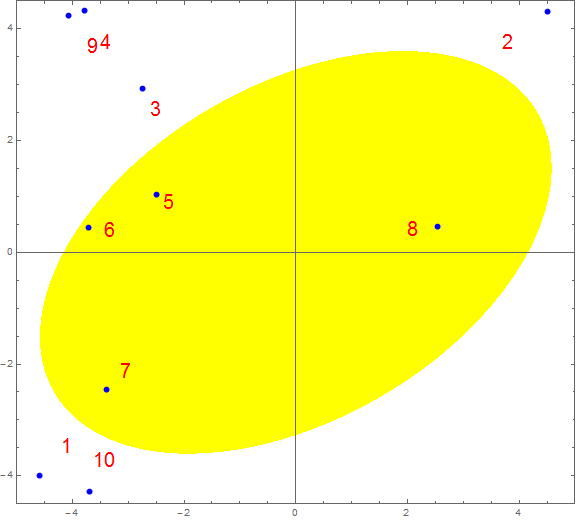卷绕数的几何意义

这个部分希望能够增加一点直观生动的内容。

一个深入点的例子

前面的例子其实不能算简单，我开始后悔没有用”圆“了。

如果说圆和椭圆的情况，都还可以通过测量到”形心距离”之类的方法——即便有些小周折，这让基于winding number 或 卷绕数的方法还是有些不太自在的——”深入点的例子“必须给常规的潜在竞争关系的方法制造足够的麻烦，或者至少要让心目中想要突出的、中意的方法干得更加漂亮！

下面这个例子，一方面仍然保持图形以及参数方程本身在视觉上或表面看上去的足够简单，同时希望让那些初级的方法更加步履维艰，否则我写了这么多不是瞎忙活吗。这条曲线的参数方程是： {x(t)=y(t)=9sin2t+5sin3t9cos2t−5cos3t<!--//--><![CDATA[//><!--
\begin{array}{rl}
x(t)=& 9  \sin 2 t+5 \sin 3 t \\
y(t)=& 9 \cos 2 t-5 \cos 3 t \\
\end{array}
\right.
//--><!]]>
把它画出来是这样的，看上去是不是有些似曾相识？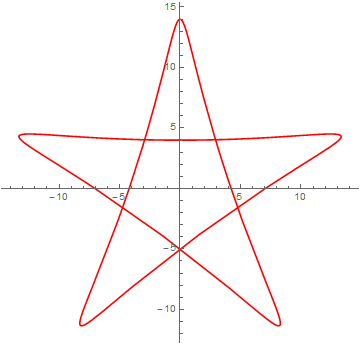在用凹多边形表述发现代码太长之后，我想尝试用曲线近似表达一颗五角星，结果就找到了它。它的边上的肌肤的线条都是C∞<!--//--><![CDATA[//><!--
C^\infty
//--><!]]>光滑的，不像凹多边形那样有棱有角；但是形状有非常近似；所有的边界都可以用一个方程表达，看上去似乎有压缩代码的潜力。如果可以简洁高效地判断出内点外点，只须再作如下填充不就是五角星了吗：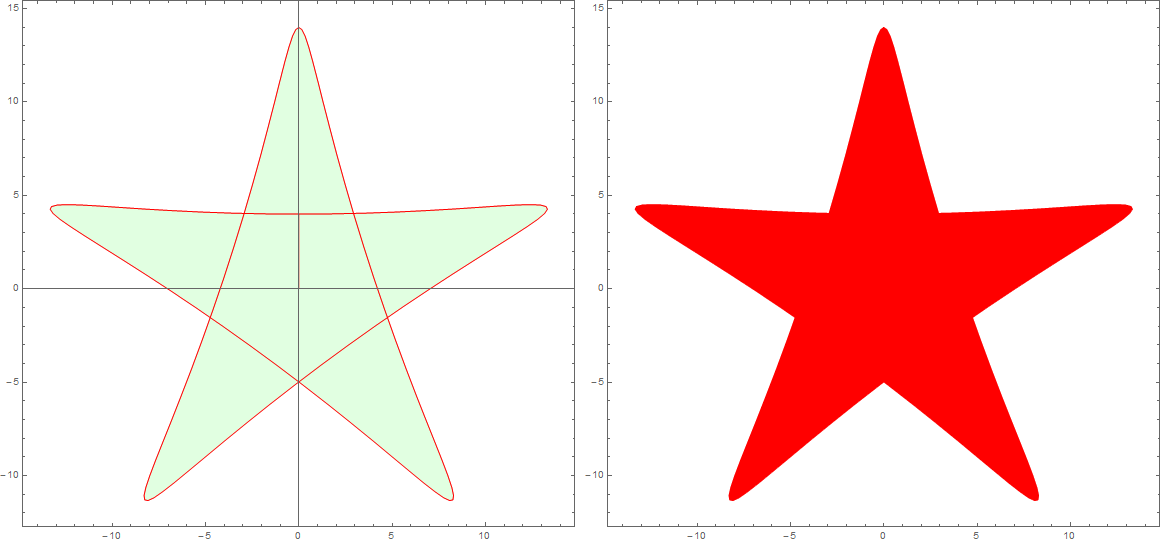代码悄悄地：

Grid@{{ParametricPlot[u {9 Sin[2 t]+5 Sin[3 t],9 Cos[2 t]-5 Cos[3 t]},{t,0,2 Pi},{u,0,1},MeshFunctions->{(*Sqrt@(#1^2+#2^2)*)#4&},Mesh->{{1}},PlotPoints->30,MeshStyle->Red,MeshShading->{{Cyan,LightGreen}},ImageSize->Large]/.Line[x_]:>{Red,Line[x]},ParametricPlot[u {9 Sin[2 t]+5 Sin[3 t],9 Cos[2 t]-5 Cos[3 t]},{t,0,2 Pi},{u,0,1},MeshFunctions->{(*Sqrt@(#1^2+#2^2)*)#4&},Mesh->{{1}},PlotPoints->30,MeshStyle->Red,MeshShading->{{Red,Red}},ImageSize->Large,Axes->False]/.Line[x_]:>{Red,Line[x]}}}

郁闷的是，对它进行判断的方法，跟椭圆的时候没有区别。所以，这部分就不讲了。连个偷着乐的表情都不能发，MarkDown太死板、不灵活。不过算法似乎可以趁机把MarkDown的流程图练习一下, 等着吧。

此外，对于这个例子为何比椭圆情况更进一步没有解释。
对winding number的解释,也缺乏维基那样生动的动态演示.
虽然不是必要的,作为练习还是可以慢慢加上来的.

向多边形的推广

惊艳的其中一部分在这里。 拓扑的确不一样。

卷绕数在五角星绘制中的应用实例

本来问题就是从绘制五星红旗开始的。为了压缩代码，希望找到一个新的判断内外点的算法。现在不论成功与否，贴代码吧。

我只把高级语言当作表达某些意思的工具，但是从来没有去研究关于这类工具的特殊的形式和技巧，无从着手，也不是特别感兴趣。所以，算法在此，进一步压缩只能翘首等别人了。

// NOTE: compile with g++ filename.cpp -std=c++11
#include <iostream>
#include <cmath>
#include <cstdlib>
#include <vector>
//#include <functional>
#define DIM1 600
#define DIM2 400
#define DM1 (DIM1-1)
#define DM2 (DIM2-1)
#define _sq(x) ((x)*(x)) // square
#define _cb(x) abs((x)*(x)*(x)) // absolute value of cube
#define _cr(x) (unsigned char)(pow((x),1.0/3.0)) // cube root

#define pi 3.14159
#define eps 2.02e-16
double arct(double x,double y){//可改写为宏放Rd函数中
return abs(x)>eps?(x>eps?atan(y/x):(y>0?atan(y/x)+pi:atan(y/x)-pi)):(y>0?pi/2:-pi/2);
}

bool inQ(int i,int j,int* px){//改为lambda表达式放GR/BL中
double art,aro;
for(int k=0;k<5;k++){
art[k]=arct((double)(px[k]-i),(double)(px[k+5]-j));//
}
double total=0.;
for(int k=0;k<5;k++){
aro[k]=art[(k+1)%5]-art[k];
while(aro[k]<-pi) aro[k]+=pi;
while(aro[k]>pi) aro[k]-=pi;
total+=aro[k];
}
return abs(total)>0.5;
};//即使修改了也只能作有限压缩，不能达到420字符水平

unsigned char GR(int,int);
unsigned char BL(int,int);

unsigned char RD(int i,int j){
return 255;
}
unsigned char GR(int i,int j){
int p1={100, 135, 43, 157, 65, 40, 149, 81, 81, 149},
p2={184, 220, 183, 207, 205, 168, 181, 191, 161, 199},
p3={183, 208, 204, 185, 220, 50, 22, 59, 27, 42},
p4={221, 259, 229, 239, 252, 135, 133, 157, 120, 156},
p5={220, 254, 237, 231, 258,83, 66, 100, 62, 89};
return inQ(i,j,p1)||inQ(i,j,p2)||inQ(i,j,p3)||inQ(i,j,p4)||inQ(i,j,p5)?255:0;
}
unsigned char BL(int i,int j){
return 0;
}

void pixel_write(int,int);
FILE *fp;
int main(){
fp = fopen("MathPic.ppm","wb");
fprintf(fp, "P6\n%d %d\n255\n", DIM1, DIM2);
for(int j=0;j<DIM2;j++)
for(int i=0;i<DIM1;i++)
pixel_write(i,j);
fclose(fp);
system("pause");
return 0;
}
void pixel_write(int i, int j){
static unsigned char color;
color = RD(i,j)&255;
color = GR(i,j)&255;
color = BL(i,j)&255;
fwrite(color, 1, 3, fp);
}


代码的输出结果ppm经过XnView或Convert转换成png格式之后是这样的：foo bar foo
foo bar foo

Edit

【稍安勿躁，正在加班加点更新】“相濡以沫，不如相忘于江湖。” 我发现庄子就像读过鲁迅的《伤逝》一样，早在几千年前就建议好了该放下的时候要果断放下，可还是有人执迷不悟。——这就是古人的大智慧。“难道我们还不如古人吗？”(后面这个反问句在我高中一同学的作文里常用，每次想起他在班里当众读自己作文里这一句时气势汹汹的样子，我都想回答“是的。在很多方面。”这也是我瞧不起一些文科生和文科公知的原因: 立论完全罔顾事实,单纯依赖辩论技巧. 你他么这样自欺欺人能解决黎曼猜想还是哥德巴赫猜想?) ↩我觉得刚才我写到这里没更新的时候,肯定有点进来的人看了很失望,因为实际上到此为止,除了画了个椭圆,我啥也没讲。会不会觉得博主不太靠谱？那一定是因为你自己更不靠谱！我觉得评价一个人靠谱与否的唯一标准是看此人是不是删过facebook  ↩“拓扑学”听上去挺抽象，但我觉得数学之所以看上去让人望而却步，除了某些基础性的基本功的确需要积累和修炼之外，大部分因为老师的方法有问题。 在深入了解这个概念之前就知道了“判断是内点或外点”时这个概念该怎么用——也就是实际使用的方法是很简单的——，不过，我边写边完善的过程中发现有更多相关而有趣的不同表述，比我原先想象的精彩得多，需要自己细细琢磨，所以更新的速度可能会变慢。学习速度更快的不妨自己先看维基。 ↩
展开全文微分几何 计算几何 算法 参数方程
• 判断是否在闭合曲线内 以前在做医疗靶区勾画时用到过这个算法，现在总结一下，介绍引射线法。 1、原理 从目标点引一条射线，判断这条线与曲线的交点个数为奇数还是偶数，为奇数表示点在曲线内，偶数表示点...
判断点是否在闭合曲线内

以前在做医疗靶区勾画时用到过这个算法，现在总结一下，介绍引射线法。

1、原理
从目标点引一条射线，判断这条线与曲线的交点个数为奇数还是偶数，为奇数表示点在曲线内，偶数表示点在曲线外。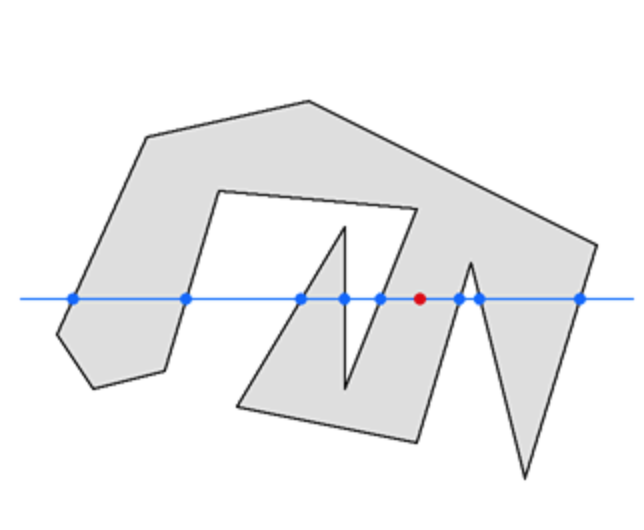js代码如下：
function pointInPolygon(point, lines) {
var inside = false;
for (var i=0; i<lines.length-1; i++) {
var slope = (lines[i+1].y - lines[i].y)/(lines[i+1].x - lines[i].x);
var xBetween = (lines[i].x < point.x && point.x <lines[i+1].x)
|| (lines[i+1].x < point.x && point.x <lines[i].x);
var yAbove = point.y < (slope * (point.x - lines[i].x) + lines[i].y);
if (xBetween && yAbove) {
inside = !inside;
}
}
return inside;
}

上面是向Y轴正方向引射线，判断射线与曲线的交点个数。
下面是github上的插件https://github.com/substack/point-in-polygon
ps: 如有意见或建议，欢迎指出


展开全文• 转自：Spline导数及曲率计算 1 概述  曲率的公式为： ... 根据参考资料的提示，可以使用csape()对离散点进行Spline插值，然后使用fnder()对得到Spline曲线求导，最后使用fnval()对导数求值即可。 [p


转自：Spline导数及曲率计算

1 概述
曲率的公式为：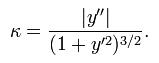因此求曲率的重点在于获得拟合曲线的一阶导数和二阶导数。
2 Matlab中的实现
2.1 实例1
根据参考资料的提示，可以使用csape()对离散点进行Spline插值，然后使用fnder()对得到Spline曲线求导，最后使用fnval()对导数求值即可。

[plain] view
plain copy%% 原数据
x = 0 : 0.1 : 2 * pi;
y = sin(x);
plot(x, y, 'o')

%% spline拟合
pp = csape(x, y);

%% 拟合图
hold on
plot( x, fnval(pp, x), 'g' );

%% 求导
pp1 = fnval(fnder(pp, 1), x); %求一阶导
pp2 = fnval(fnder(pp, 2), x); %求二阶导

%% 曲率，K = |y''| / ((1 + y'^2)^(3/2))
curvature = abs(pp2) ./ sqrt( (1 + pp1 .^ 2) .^ 3 );

%% 曲率图
hold on
plot( x, curvature, 'r' );

%% 图例
legend('原始散点', 'Spline拟合结果', '曲率图');

%% 显示网格
grid on
上述代码的运行结果如下图所示：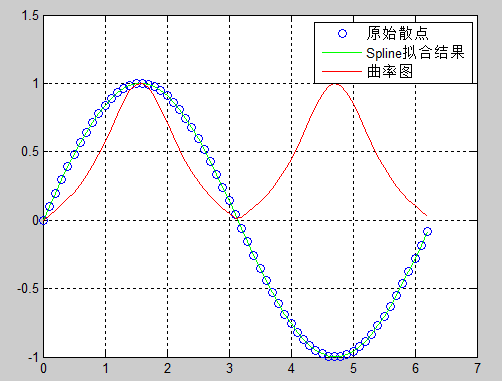2.2 实例2
参考资料给出了另外一个实例：

[plain] view
plain copy%% 原始数据点
xx=[-1  0  3  5  8  9 ];
yy=[1  7  -4  0  7  3  ];    %给定6个点

%% spline拟合
sp = spline(xx,yy);    % spline(x,y)  曲线插值

x = xx(1):0.01:xx(length(xx));      %通常取x(1)至x(n)
y = ppval(x,sp);                     % ppval( , )   分段多项式的值  y的值变为数组y（）

subplot(311)
for i=1:length(xx)
plot(xx(i), yy(i),'b*')
hold on
end
plot(x,y,'r');
grid on
title('B样条插值曲线')

%% 斜率计算
for i=1:(length(x)-1)
dx(i)=x(i+1)-x(i);
dy(i)=y(i+1)-y(i);              % 离散一次导（相当于连续一次导数）
dddy(i)= dy(i)/dx(i);
end

%% 曲率计算
for i = 1 : (length(x)-2)
ddx(i) = dx(i+1) - dx(i);
ddy(i) = dy(i+1) - dy(i);      % 离散二次差分(相当于连续二次导)
K(i)=(dx(i)*ddy(i)-dy(i)*ddx(i))/((dx(i)*dx(i)+dy(i)*dy(i))^1.5); % 曲率
end

subplot(312)
ud=linspace(xx(1),xx(length(xx)),(length(x)-1));
plot(ud,dddy)
grid on
title('曲线斜率变化图')

subplot(313)
uu=linspace(xx(1),xx(length(xx)),(length(x)-2));
plot(uu,K)
grid on
title('曲线曲率变化图')

上述代码执行效果如下图：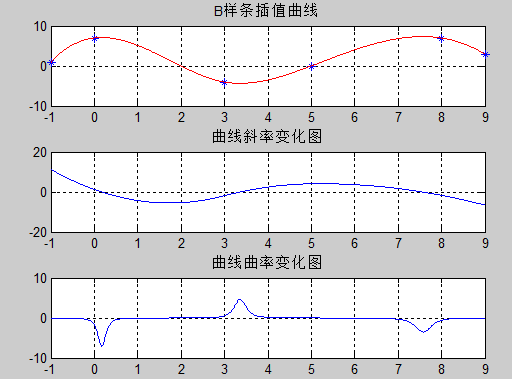参考资料
三次B样条曲率与导数算法Matlab代码
样条曲线
B样条曲线曲率简易求解算法
如何用matlab提取曲线各点曲率
如何得到matlab三次样条曲线插值（csape）之后得到的拟合曲线的曲率图
用matlab求出最小曲率半径曲线方程实例
对离散样点做三次样条曲率计算和求导的matlab程序
知道一些坐标，怎么用matlab画出曲线并计算出曲线的曲率
计算曲率
用MATLAB求曲线在某一点的曲率
对给定的离散点，先拟合成B样条曲线，再求B样条曲线曲率
Curvature of a spline
Curvature of Approximating Curve Subdivision Schemes

顶
0
踩

展开全文• ## ROC曲线详解

万次阅读 2014-10-21 15:37:02
ROC曲线指受试者工作特征曲线 / 接收器操作特性曲线(receiver operating characteristic curve), 是反映敏感性和特异性连续变量的综合指标,是用构图法揭示敏感性和特异性的相互关系，它通过将连续变量设定出多个不同...
        ROC曲线指受试者工作特征曲线 / 接收器操作特性曲线(receiver operating characteristic curve), 是反映敏感性和特异性连续变量的综合指标,是用构图法揭示敏感性和特异性的相互关系，它通过将连续变量设定出多个不同的临界值，从而计算出一系列敏感性和特异性，再以敏感性为纵坐标、（1-特异性）为横坐标绘制成曲线，曲线下面积越大，诊断准确性越高。在ROC曲线上，最靠近坐标图左上方的点为敏感性和特异性均较高的临界值。

1 ROC曲线的例子

考虑一个二分问题，即将实例分成正类（positive）或负类（negative）。对一个二分问题来说，会出现四种情况。如果一个实例是正类并且也被 预测成正类，即为真正类（True positive）,如果实例是负类被预测成正类，称之为假正类（False positive）。相应地，如果实例是负类被预测成负类，称之为真负类（True negative）,正类被预测成负类则为假负类（false
negative）。

列联表如下表所示，1代表正类，0代表负类。

预测

1

0

合计

实际

1

True Positive（TP）

False Negative（FN）

Actual Positive(TP+FN)

0

False Positive（FP)

True Negative(TN)

Actual Negative(FP+TN)

合计

Predicted Positive(TP+FP)

Predicted Negative(FN+TN)

TP+FP+FN+TN

从列联表引入两个新名词。其一是真正类率(true positive rate ,TPR), 计算公式为TPR=TP/ (TP+ FN)，刻画的是分类器所识别出的 正实例占所有正实例的比例。另外一个是假正类率(false
positive rate, FPR),计算公式为FPR= FP / (FP + TN)，计算的是分类器错认为正类的负实例占所有负实例的比例。还有一个真负类率（True Negative Rate，TNR），也称为specificity,计算公式为TNR=TN/
(FP+ TN) = 1 - FPR。

在一个二分类模型中，对于所得到的连续结果，假设已确定一个阀值，比如说 0.6，大于这个值的实例划归为正类，小于这个值则划到负类中。如果减小阀值，减到0.5，固然能识别出更多的正类，也就是提高了识别出的正例占所有正例 的比类，即TPR,但同时也将更多的负实例当作了正实例，即提高了FPR。为了形象化这一变化，在此引入ROC，ROC曲线可以用于评价一个分类器。ROC曲线和它相关的比率

(a)理想情况下，TPR应该接近1，FPR应该接近0。

ROC曲线上的每一个点对应于一个threshold，对于一个分类器，每个threshold下会有一个TPR和FPR。

比如Threshold最大时，TP=FP=0，对应于原点；Threshold最小时，TN=FN=0，对应于右上角的点(1,1)

(b)P和N得分不作为特征间距离d的一个函数，随着阈值theta增加，TP和FP都增加

Receiver Operating Characteristic,翻译为"接受者操作特性曲线"，够拗口的。曲线由两个变量1-specificity 和 Sensitivity绘制. 1-specificity=FPR，即负正类率。Sensitivity即是真正类率，TPR(True positive rate),反映了正类覆盖程度。这个组合以1-specificity对sensitivity,即是以代价(costs)对收益(benefits)。

此外，ROC曲线还可以用来计算“均值平均精度”（mean average precision），这是当你通过改变阈值来选择最好的结果时所得到的平均精度（PPV）。

2 ROC曲线下的面积

在比较不同的分类模型时，可以将每个模型的ROC曲线都画出来，比较曲线下面积做为模型优劣的指标。

ROC曲线下方的面积（英语：Area under the Curve of ROC
(AUC ROC)），其意义是：
因为是在1x1的方格里求面积，AUC必在0~1之间。假设阈值以上是阳性，以下是阴性；若随机抽取一个阳性样本和一个阴性样本，分类器正确判断阳性样本的值高于阴性样本之机率$=AUC$。简单说：AUC值越大的分类器，正确率越高。
从AUC判断分类器（预测模型）优劣的标准：
AUC = 1，是完美分类器，采用这个预测模型时，存在至少一个阈值能得出完美预测。绝大多数预测的场合，不存在完美分类器。0.5 < AUC < 1，优于随机猜测。这个分类器（模型）妥善设定阈值的话，能有预测价值。AUC = 0.5，跟随机猜测一样（例：丢铜板），模型没有预测价值。AUC < 0.5，比随机猜测还差；但只要总是反预测而行，就优于随机猜测。

Reference：
http://blog.csdn.net/abcjennifer/article/details/7359370
`
展开全文ROC 机器学习评价
• 如何判断轮廓是否为圆？ 如何判断轮廓是否为圆？ 判断一个轮廓是否为圆？这看似简单的问题，在opencv中并没有现成的函数。当我真正想运用的时候，却发现还是有许多内容在里面的。 比如这幅图片，由于瓶口...
• ## 曲线拟合

万次阅读 2015-05-01 00:40:48
曲线拟合(Curve Fitting)的数学定义是指用连续曲线近似地刻画或比拟平面上一组离散点所表示的坐标之间的函数关系，是一种用解析表达式逼近离散数据的方法。曲线拟合通俗的说法就是“拉曲线”，也就是将现有数据...数据 算法
• ## ROC曲线学习总结

万次阅读 多人点赞 2019-08-19 15:22:38
文章目录ROC曲线学习总结1. ROC曲线(Receiver Operating Characteristic)的概念和绘制2. 利用ROC曲线评价模型性能——AUC(Area Under Curve)3. 利用ROC曲线选择最佳模型3.1 不同模型之间选择最优模型3.2 同一模型中...
• 博主声明： 转载请在开头附加本文链接及作者信息，并... 直接步入正题，我们要实现的是一个 Android 客户端应用里面的一种点赞效果，比如你点一下那个爱心型的图片，就会产生一个小爱心，而且会以曲线的方式进行上...Bezier
• # 将每次运行的KS值存入一个文件，每次运行时加入文件，再读取文件画出连续一些天数的ks曲线图  if os.path.exists('./ks_accumulate.csv'):  if os.path.getsize('./ks_accumulate.csv'):  print('文件...
• ## ROC曲线

千次阅读 2014-03-11 17:14:58
转载：百度百科 ...在做算法性能分析中，通常会与其它方法进行对比，而目前常用的曲线方法是ROC曲线，它能直观的显示出算法性能的优劣。 受试者工作特征曲线 （receiver operating chara性能分析
• 0x00 需求的诞生 在上一个版本的开发过程中，美术同事提出了按照《悬空城》的活动列表...1.如何构造出列表的曲线，其实曲线就是其中的每个元素所在的x坐标不同，从纵向的角度看构成了一条连续曲线。 2.何时刷新列表
• ## 贝塞尔曲线

千次阅读 2017-12-09 09:44:01
贝塞尔曲线 什么是贝塞尔曲线 贝塞尔曲线(Bézier curve)，又称贝兹曲线或贝济埃曲线，是应用于二维图形应用程序的数学曲线。一般的矢量图形软件通过它来精确画出曲线，贝兹曲线由线段与节点组成，节点是可...
• 曲线拟合(Curve Fitting)的数学定义是指用连续曲线近似地刻画或比拟平面上一组离散点所表示的坐标之间的函数关系，是一种用解析表达式逼近离散数据的方法。曲线拟合通俗的说法就是“拉曲线”，也就是将现有数据透过...算法 c++ 数据 数学计算 技术
• 曲线曲面基本理论一 、曲线曲面基本理论 计算机图形学三大块内容：光栅图形显示、几何造型技术、真实感图形显示 。光栅图形学是图形学的基础，有大量的思想和算法 。几何造型技术是一项研究在计算机中，如何表达...
• 一、使用图形对数据初步进行描述。...常用直方图，适用于连续性数据。 hist(x),lines(density(x)) 2.经验分布图，一般的总体分布。 ecdf(x) #生成x的向量 plot(x, ..., ylab="Fn(x)", vert...r语言
• 二次 贝塞尔曲线 和三次 贝塞尔曲线 使用说明 1. 二次 贝塞尔曲线 和三次 贝塞尔曲线1.1 贝塞尔曲线的 基本知识1.2 贝塞尔曲线 生成动图1.3 HTML 中 画贝塞尔曲线的 2 种方法1.4 二次 贝塞尔曲线 使用1.4.1 二次 ...html标签 canvas标签
• ## 椭圆曲线介绍

千次阅读 2014-04-13 21:50:28
• ROC曲线指受试者工作特征曲线 / 接收器操作特性曲线(receiver operating characteristic curve), 是反映敏感性和特异性连续变量的综合指标,是用构图法揭示敏感性和特异性的相互关系，它通过将连续变量设定出多个不同...ROC
• 基于echarts实现根据鼠标移动轨迹更改曲线值或创建曲线 ** 有不懂的，可以留言哈， <!DOCTYPE html> <html style="height: 100%"> <head> <meta charset="utf-8"> </head>...echarts
• 上篇已经整理了一下Android 的基本动画（Android 动画-简介），这次来梳理一下贝塞尔曲线的用法。 说道贝塞尔曲线，应该想到的是自定义view，确实自定义view是绘制贝塞尔曲线的基础，绘制使用好贝塞尔曲线带来的...android 动画
• ## 缓和曲线——回旋曲线的计算

千次阅读 多人点赞 2020-05-12 23:12:49
缓和曲线指的是平面线型中，在直线与圆曲线、圆曲线与圆曲线之间设置的曲率连续变化的曲线。缓和曲线的线型多种多样，如回旋线、三次抛物线、七次四项式型、半波正弦型、一波正弦型、双纽线、多心复曲线…… 铁路上...matlab 算法 自动驾驶
• ## 判断一个点是否在多边形内部

万次阅读 多人点赞 2015-04-10 16:23:22
一、比如说，我就随便涂了一个多边形和一个点，现在我要给出一种通用的方法来判断这个点是不是在多边形内部（别告诉我用肉眼观察……）。 首先想到的一个解法是从这个点做一条射线，计算它跟多边形边界的交点...算法 多边形
• （1）绘制余弦曲线和直线 /* 绘制余弦曲线和直线 在屏幕上显示0~360度的cos(x)曲线与直线f(x)=45*y+31的迭加图形。...图形迭加的关键是要在分别计算出同一行中两个图形的列方向点坐标后，正确判断相互的
•  ROC 曲线指受试者工作特征曲线 (receiver operating characteristic curve), 是反映敏感性（正确判断病人概率）和特异性（正确判断非病人概率）连续变量的综合指标, 是用构图法揭示敏感性和特异性的相互关系，它...数据分析
• 判断一个点是否在多边形内部 - 射线法思路 比如说，我就随便涂了一个多边形和一个点，现在我要给出一种通用的方法来判断这个点是不是在多边形内部（别告诉我用肉眼观察……）。 首先想到的一个解法是从这个点做一条...
• 前端时间公司项目中有用到Bezier曲线的知识，在熟悉Bezier曲线原理和实现方式后，我突发奇想在Android客户端实现Bezier曲线的构建动画，于是有了BezierMaker这个项目。在讲解代码前，我们先了解一下Bezier曲线的原理...android Bezier 德卡斯特里奥算法...